﻿ Development of Learning Devices Based on Metacognition Approach to Improve Mathematical Communication Ability of Students in State SMP 30 of MedanPublications are Open
Access in this journal
Article Versions
Export Article
• Normal Style
• MLA Style
• APA Style
• Chicago Style
Research Article
Open Access Peer-reviewed

### Development of Learning Devices Based on Metacognition Approach to Improve Mathematical Communication Ability of Students in State SMP 30 of Medan

Eka Rezki Nopianty, Kms. Muhammad Amin Fauzi, Mukhtar
American Journal of Educational Research. 2018, 6(11), 1465-1472. DOI: 10.12691/education-6-11-4
Received October 02, 2018; Revised November 06, 2018; Accepted November 12, 2018

### Abstract

This study aims to: 1) Describe validity, practicality, and effectiveness of learning devices based on metacognition approach developed on mathematical communication ability of students in State SMP 30 of Medan; 2) Describe the improvement of mathematical communication ability using learning devices based on the metacognition approach developed. Researchers develop learning devices based on a metacognition approach using the Dick & Carey development model. The learning devices produced from this study are the teacher’s book and student’s books. From the results of this study it was found that: 1) the developed learning devices fill the criteria of valid, practical, and effective in terms of their respective criteria; 2) in the first trial there was an increase in mathematical communication ability using learning devices developed in the low category, it’s about 0.28 and in the second trial there was an increase in mathematical communication ability using learning devices developed in the medium category, it’s about 0.56.

### 1. Introduction

Education is one of the national development sectors in an effort to educate the lives of the nation in order to produce quality human beings. Through the learning process, it is hoped that national education can function optimally as the main vehicle for nation building and character building. According to Tirtarahardja 1 education is one of the main pillars in anticipating the future, because education is always oriented towards preparing students to play a role in the future. The role of education in the future is closely related to learning mathematics.

The aim of mathematics subjects in schools for elementary and secondary education levels is so that students can: (1) use patterns as a guess in solving problems and be able to make generalizations based on existing phenomena or data; (2) using reasoning in characteristic, manipulating mathematics both in simplification, and analyzing existing components in problem solving; (3) communicating ideas, reasoning, and compiling mathematical evidence using complete sentences, symbols, tables, diagrams, or other media to clarify the situation or problem.

One of the things that must be considered in carrying out mathematics learning so that the objectives of mathematics learning can be achieved is learning devices. This is consistent with the opinion of Haggarty and Keynes (in Muchayat) 2 that in order to improve the teaching and learning of mathematics in the classroom it takes effort to improve the understanding of teachers, students, and devices used for learning and interaction between them. In line with Aunurrahman 3 which said that during the learning process takes place, learning problems are often related to learning devices and learning resources. Referring to this, it is clear that learning devices play an important role in the successful implementation of the learning process.

The development objective of the 2013 Curriculum conducted in Indonesia is to change the learning process from students who are told to be students who find out, the assessment process from being output-based to process-based and output, as well as balancing soft skills and hard skills. One of the skills demanded in the 2013 curriculum and 21st century competencies must be built is mathematical communication ability. This is in line with one of the objectives of learning mathematics stated in Permendikbud Number 58 of 2014, that students must be able to communicate ideas, reasoning, and compile mathematical evidence by using complete sentences, symbols, tables, diagrams, or other media to clarify the situation or problem .

To realize these goals, of course a learning devices must be developed and of course a learning approach that fits the student’s ability to achieve, of course, is an approach that encourages students to construct knowledge in their own minds so that their unfavorable influence on the development of student’s mathematical ability does not continue to negative attitude towards mathematics. One way that can be used is with learning devices based on metacognition approach.

The metacognition approach is one of the learning approaches that can encourage students to construct mathematical ideas in solving mathematical problems. Learning devices developed are based on metacognition approaches for several reasons. First, one of the learning processes that is believed to improve students' mathematical communication ability is learning based on a metacognition approach. Second, based on research conducted by Hassan and Ahmed 4 it is said that the metacognition approach has a high influence in improving student academic achievement. Then, Partanen, Jansson, Lisspers, and Sundin 5 said that the metacognition approach consists of a monitoring strategy that is used in different stages of learning, from planning to completing a task, making a summary, and evaluating after completing the task. Third, based on research conducted by Piadi 6 that the metacognition approach is an approach that makes students aware of the advantages and limitations of learning. This means that when students know their mistakes, they realize then correct them and immediately realize how they should be, and direct the student’s attention to the relevant matters and guide them to choose suitable strategies in solving problems through questions. With metacognition, students are not only skilled at understanding the sequence of work, but they are aware of the process carried out in solving mathematical problems. The results of the research by Rizqi, Asmin, and Fauzi 7 also found that there was an increase in students' mathematical reasoning ability through learning devices based on the developed metacognition approach.

Based on the description, it is expected that the development of mathematics learning devices based on metacognition approaches can improve students' mathematical communication ability.

### 2. Research Method

This research is a development research with the Dick & Carey development model 8 which consists of 10 stages of development. The whole stage of developing the Dick & Carey model is shown in Figure 1 below.

2.1. Subjects and Research Objects

Subjects in this study were students of class VII-6 and VII-8 in State SMP 30 of Medan in the 2018/2019 academic year, each of which amounted to 32 students. While the objects in this study are learning devices based on metacognition approach on social arithmetic, namely Teacher Guidelines (BPG) and Student Books (BS).

2.2. Data Analysis Instruments and Techniques The

Instruments used in this study are instruments to measure validity, practicality, and effectiveness of the learning devices developed, namely validation sheets, observation sheets, tests, and questionnaires.

• Figure 1. Dick & Carey Development Model

2.3.1. Validity of Learning Devices

Learning devices are validated to experts namely five validators by giving a score of 1 to 5 in each column of assessment based on aspects: (1) format, (2) language, (3) content, and (4) illustration. Furthermore, the overall expert judgment is processed by calculating the average score to get the validity assessment criteria as follows:

Learning devices based on metacognition approach fill the expected validity if the average validator rating of all valid or very valid learning devices. If it has not been reached, it needs to be validated again until valid. Furthermore, the student’s mathematical communication ability test instrument was tested outside the sample class to measure the validity and reliability of the instrument. The validity of the item is calculated using thecorrelation formula Product Moment 9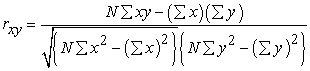(1)

Description: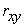: The coefficient between variables x and y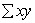: The number of multiplication results between x and y

x: The acquisition score of item

y: Total score

N: Number of students

The reliability coefficient of the question is calculated using theformula Alpha-Cronbach 10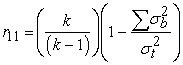(2)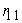: test reliability coefficient

k: Number of item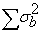: Number of variance test scores of each item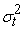: Total variance

2.3.2. Practicality Learning Devices

Herman 11 said that learning devices are said to be practical if according to the assessment of experts and practitioners the learning devices are stated to be applicable and observations of the implementation of learning devices in the class are included in good or very good categories.

2.3.3. Effectiveness of Effectiveness Learning Devices

In learning devices in this study were reviewed based on: (1) classical student learning outcomes; (2) student’s positive response of learning devices; and (3) the ability of teachers to manage learning.

2.3.4. Increased Mathematical Communication Ability

The amount of mathematical communication ability improvement is calculated by theformula N-gain of Hake 13 as follows: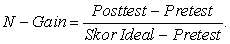(3)

With the gain index criteria as in the following table:

### 3. Research Results

3.1. Description of the Development Phase of Learning Devices

Development of learning devices has been completed using the Dick & Carey development model with the following details.

3.1.1. The Identification of Teaching Objectives

The purpose of teaching can be identified by first analyzing instructional needs. The process of identifying instructional needs begins with identifying problems in the field. Based on the tests given, it was concluded that student’s mathematical communication ability were still low. The results of observations and interviews with teachers and students show that the cause is that students are not accustomed to communicating mathematical ideas in learning activities. This is also supported by the condition of teachers who have not compiled learning devices that focus on developing students' mathematical communication ability. Based on these findings, the main objective in developing this teaching material is to develop and improve students' mathematical communication ability.

3.1.2. Conducting Teaching

Analysis Instructional analysis in this study is a process of describing general competencies into sub-competencies, basic competencies or special sub-competencies that are arranged logically and systematically. The activity is intended to identify a list of sub-competencies and compile relationships between one another towards general competencies in the 2013 curriculum called core competencies. The material to be taught is social arithmetic. So that the basic competencies of the material are outlined, and indicators are developed to achieve success.

3.1.3. Student Analysis

Cognitive development of research subjects in State SMP 30 of Medan entered the formal operational stage because it was in the age range of 12-15 years. The stage is marked by a more logical, abstract, and idealistic way of thinking. Target subjects in grade VII are students who are quite heterogeneous when viewed with cognitive abilities. Cooperative learning with group discussion is also still rarely done and students also have never trained in mathematical communication ability in solving problems.

3.1.4. The Formulating Learning Objectives

The formulation of learning objectives is based on indicators to achieve basic competencies that have been set aside in the second development stage. The learning objectives in this study are based on indicators of the basic competencies of social arithmetic material.

3.1.5. Developing Instruments

At this stage a learning outcome test is developed to measure student’s mathematical communication ability. Tests are prepared based on the learning objectives to be achieved. Based on these objectives 4 essay tests were arranged and each question included indicators of mathematical communication ability.

3.1.6. Developing Teaching Strategies

At this stage an approach will be identified that will be used to achieve the objectives. The learning approach used is a metacognition approach. This metacognition approach consists of three stages, namely: planning, monitoring, and evaluating. The three stages are reflected and arranged in the form of learning implementation plans (RPP) which are arranged in 4 sets for 4 meetings. The RPP covers all descriptions of activities that will be carried out at each meeting.

3.1.7. Developing and Selecting Learning Devices

The main activities in this phase are the initial drafting of learning materials which include the teacher's book and student’s book. Learning devices that are arranged are targeted at KI, KD, and indicators on social arithmetic material, and are adjusted to the purpose to train and improve student’s mathematical communication ability.

3.1.8. Implementing Formative Evaluation

At this stage an evaluation of the learning tools that have been developed. Formative evaluation is carried out in 2 stages, namely: 1) evaluation one by one by experts and practitioners, and 2) field trials. The aim is to see weaknesses and improve learning devices that have been developed.

One by one evaluation results by experts and practitioners in the form of an assessment of content validity indicate that all learning devices fill valid criteria, with an average validity content of RPP = 4.22; LKS = 4.33; BPG (teacher’s book) = 4.24; and BS (student’s book) = 4.31. All mathematical communication ability test items fill valid and reliable criteria with r11 = 0.652 (high category) for pretest and r11 = 0.700 (high category) for posttest.

Field trials or trial I were carried out to see the practicality and effectiveness of learning devices. In the first trial, the instructional material did not fill all the effective criteria, so it had to be revised to the learning devices and the second trial was carried out again.

3.1.9. The Revision Teaching

Revision was carried out based on the findings of the weaknesses of the learning devices in the trial I. The revision was carried out on the teacher’s book and the student’s book related to the material being taught. After the revision is complete, an advanced field trial or trial II is conducted to review the practicality and effectiveness of learning devices, as well as the improvement of student’s mathematical communication ability at each trial.

3.1.10. Conduct Summative Evaluation Sumative

Evaluation in this study was not carried out. This is because summative evaluation is not part of the instructional design process. Formative evaluation and summative evaluation cannot be coupled as two successive activities, but interspersed with the implementation period so that it takes a long time and costs a lot to do it 14.

3.2. The Description Trial Result I

Criteria for practicality of learning devices based on validator assessment were fulfilled, because all validators assessed the developed learning devices could be used with "little revision" or "without revision". The fulfillment of learning devices is fulfilled, in terms of the average of all learning meetings obtaining a percentage of 80.01% (good category). Based on this description, the instructional material developed fill the practical criteria.

The test results of student’s mathematical communication ability classically show the number of subjects who complete a score of ≥ 75 reaching 25 students from 32 students or 78.12% so that they do not fill the expected criteria of completeness of classical learning outcomes. Criteria for effectiveness based on student responses have been fulfilled, because students who provide a positive response to the components and implementation of learning reach mencapai 80% that is 86.64%. Furthermore, the effectiveness criteria based on the teacher's ability to manage learning are fulfilled because they reach an average value of 4.25 (good category). Overall learning devices developed fill the valid and practical criteria, but have not met the criteria for effectiveness. This is because the completeness aspect of student learning outcomes is not yet fulfilled classically. Thus the teaching material must be revised and then carried out trial II.

From the results of the analysis of the trial data I found that the instructional material developed was not effective, because there were still indicators of effectiveness that had not been achieved. In the first trial the percentage of classical completeness achieved has not met the criteria for achieving classical completeness.

• Figure 2. Classical Completeness Percentage of Students 'Mathematical Communication Ability in Trial I

Based on the picture above it can be seen that the classical completeness of the results of student’s mathematical communication ability at pretest I test was 31.25% while in the posttest I test was 78.12%. In accordance with the criteria of classical student learning completeness, which is at least 85% of students who participate in learning are able to achieve a score of ≥ 75, then the results posttest of mathematical communication ability in test I have not met the criteria for achieving classical completeness because it only obtains the percentage of completeness 78.12%.

The indicators of effectiveness that have been fulfilled in test I are: (1) student response, that’s of students respond positively to the components of learning devices based on metacognition approach developed with the average percentage of total positive response of students in the first trial of 86.64%; and (2) the ability of teachers to manage learning, which is in a good category with an average value of a total of four meetings is 4.25.

3.3. Improvement of Student’s Mathematical Communication Ability in Trial I

Improvement of student’s mathematical communication ability in trial I was saw through N-Gain from the results pretest and posttest of mathematical communication ability in trial I. Here is a summary ofresults N-Gain based on predetermined improvement categories.

Based on Table 4 it can be seen that there are no students who experience an increase in mathematical communication ability with the category “High”. For students who experienced an increase in mathematical communication ability with the category "Medium" amounted to 14 students (43.75%), while students who experienced an increase in mathematical communication ability with the category “Low” amounted to 18 students (56.25%).

The results of N-Gain students when reviewed based on the average score of pretest and posttest mathematical communication ability also increased. The average pretest and posttest in trial I were 63.41 and 73.82 respectively, which resulted in the difference between pretest and posttest scores of 10.41. Based on the results of the N-Gain calculation, it was found that the improvement of students' mathematical communication ability in the first trial was 0.28 or in the "Low" category.

3.4. Description of Trial Result II

Criteria for practicality of instructional materials based on validator assessment are fulfilled in accordance with the description in trial I. The implementation of learning devices in trial II is also fulfilled, in terms of the average of all learning meetings obtaining a percentage of 84.35% (good category). Based on this description, the instructional material developed fill the practical criteria.

The results of the classical mathematical communication ability test showed that the number of subjects who completed a score of ≥ 75 reached 28 students from 32 students or 87.50%. So that it has met the expected criteria for completeness of classical learning outcomes. The effectiveness criteria based on student responses were also fulfilled, because students who gave a positive response to the components and learning implementation reached ≥ 80%, which is 91.96%. Furthermore, the effectiveness criteria based on the teacher's ability to manage learning are fulfilled because they reach an average score of 4.53 (very good category). So that overall learning devices based on the metacognition approach developed have met the criteria for valid, practical and effective.

• Figure 3. Classical Completeness Percentage of Students 'Mathematical Communication Ability in Trial II

Based on the picture above, it can be seen that the classical completeness of the results of students' mathematical communication ability at the pretest II trial was 34.37% while in the posttest II test was 87.50%. In accordance with the criteria of classical student learning completeness, which is at least 85% of students who take learning can achieve a score of ≥ 75, then the results of posttest mathematical communication ability in trial II have fill the criteria for achieving classical completeness.

3.5. Improvement of Student’s Mathematical Communication Ability in Trial II

Improvement of student’s mathematical communication ability in trial II was saw through N-Gain from the results of pretest and posttest mathematical communication ability in trial II. The following is a summary of the results of N-Gain based on the increase categories that have been set.

Based on Table 5 it can be seen that there are 6 students (18.75%) who experienced an increase in mathematical communication ability with the category "High". For students who experienced an increase in mathematical communication ability with the category "Medium" amounted to 22 students (689.75%), while students who experienced an increase in mathematical communication ability with the category "Low" amounted to 4 students (12.50%).

The results of N-Gain students when reviewed based on the average score of pretest and posttest mathematical communication ability also increased. The average pretest and posttest in trial I were 65.58 and 84.50 respectively, which resulted in the difference between pretest and posttest scores of 18.92. Based on the results of the N-Gain calculation, it was found that the increase in students' mathematical communication ability in the first trial was 0.56 or in the "Medium" category.

3.6. Improvement of Student’s Mathematical Communication Ability Viewed from Each Indicator

Improvement of student’s mathematical communication ability for each indicator is seen from the average acquisition of posttest scores for each indicator of mathematical communication ability in the first trial and trial II. The indicators of mathematical communication abilityare: (1) explain the idea or situation of an image, graph, diagram, or table given in their own words in writing (writing); (2) states a situation with pictures, graphs, diagrams, or tables (drawing); and (3) state the situation into a mathematical model (mathematical expression).

Based on Figure 4 and Table 6 shows that indicator 1, which explains the idea or situation of an image, graph, diagram, or table given in their own words in the form writing (writing) is an indicator that has the highest increase, it is about 0,50, and indicator 3, which states the situation into a mathematical model (mathematical expression) is an indicator that has the lowest increase, it is about 0,33.

The results obtained are supported by examples of student’s answers to mathematical communication ability test.

• Figure 4. Improvement of Student’s Mathematical Communication Ability for Each Indicator
• Figure 5. Example of Student’s Answers in Trial I
• Figure 6. Example of Student’s Answers in Trial II

Figure 5 and Figure 6 are one example of student’s answers to mathematical communication ability test, with point (a) for writing indicator, point (b) for mathematical expression indicator, and (c) for drawing indicator.

From the two images, it can be seen that students can solve problems for writing indicator, while for indicator of mathematical expression, student in trial I is still unable to solve them. Likewise for the drawing indicator, student in the first trial were able to describe the situation from a problem but could not clearly define the picture. While students in the second trial were able to make mathematical modeling and describe the situation of a problem so that they could solve the problem as expected.

### 4. Discussion of Research Result

The results showed that learning devices based on metacognition approaches developed met the criteria of valid, practical, and effective. These results indicate that the instructional material developed has good quality learning devices because it fills the criteria of valid, practical, and effective 15. After producing good quality learning devices, the next goal is the application of instructional materials developed to improve student communication ability.

Metacognition is thinking about thinking 16. According to Safari and Meskini 17 that learning processes that require planning, application, monitoring, regulation and reflection are included in the domain of metacognition. Metacognition is knowledge of one's ability to control the thinking process in solving the problem 18. Metacognition include skills learners to understand the cognitive processes 19.

In this study, each stage of the metacognition approach in learning poses questions of metacognition. According to Ozcan and Erktin 20, “cognitive questions can be incorporated into homework assignments to implement new methods in classrooms”. It means, can avoid problems when applying new methods of learning.

Based on research conducted by Hassan and Ahmed, said that the metacognition approach has a high effect in improving student’s academic achievement in line with this research, which is able to improve communication ability mathematically using students learning devices based on metacognition approach because the metacognition approach involves the ability of students to understand how students think, imagine something in their minds, and control what they learn based on understanding and the results of reflection of their thoughts.

Based on the researchers initial findings in the field related to the research subject, student’s overall mathematical communication ability were still low. This is because the teacher does not have good and appropriate learning devices for improving student’s mathematical communication ability. Haggarty and Keynes (in Muchayat) 2 said that “in order to improve the teaching and learning of mathematics in the classroom, efforts are needed to improve the understanding of teachers, students, materials used for learning and interaction between them”. These results are supported by research by Ritonga, Surya, and Syahputra 21 which provide results that by developing learning tools that fill the criteria for effectiveness of a learning device can improve students' mathematical problem solving abilities. Devesh & Nasseri's study 22, entitled: "Effectiveness of Mathematics Module in Foundation Program in Majan College", also states that many students find it easier, more useful and more interesting to learn mathematics because they use modules and the use of mathematics modules is very effective in learning.

In line with the research conducted by Fitriani, Hasratuddin and Syahputra 23, the results show that by developing learning tools that fill the effectiveness criteria of a learning device can improve students' mathematical communication ability. This is also supported by the results of research by Jamiah, Syahputra, and Fauzi 24 that by applying learning devices that involve active students in learning can improve students' mathematical communication ability. The results of the study stated that student’s mathematical communication ability with medium category were based on the calculation of N-Gain values. Therefore, in this study it can be concluded that learning devices based on metacognition approaches are developed according to the needs of students, and are able to direct and familiarize students to be able to have mathematical communication ability in solving mathematical problems.

### 5. Conclusions

Based on the results of the analysis and discussion in this study, several conclusions are presented as follows:

a) Learning devices based on the metacognition approach developed fill valid criteria. Validity fills the criteria for content validity and construct validity specified.

b) Learning devices based on the metacognition approach developed fill practical criteria. Practical criteria in terms of: (1) validator's assessment of teaching materials can be used easily, (2) students and teachers state that the instructional materials developed are easy to use, and (3) the implementation of teaching materials in learning reaches 84.35% with good categories .

c) Teaching materials based on the metacognition approach developed fill the effective criteria. Effective criteria in terms of: (1) completeness of student learning outcomes classically with a minimum score of 75 has been achieved 87.50%, (2) positive response of students reaches 91.96% of the components of teaching materials and learning activities, and (3) the ability of teachers to manage learning is in a very good category.

d) Mathematical communication ability of students after using teaching materials based on metacognition approach increased with a low category in trial I, which is equal to 0.28 and after the revised teaching material, students' mathematical communication ability increased with the medium category in trial II, which is equal to 0.56. The increase was reviewed based on the acquisition of N-Gain scores of students from the pretest and posttest scores in each trial.

### 6. Suggestions

Based on the results of the research and the conclusions above, it can be suggested as follows:

a) Teaching material based on the developed metacognition approach has fulfilled the aspects of validity, practicality, and effectiveness, so it is suggested to teachers in Medan 30 Public Middle School to be able to use This teaching material is used to develop students' mathematical communication ability, especially for grade VII students. However, the teacher and students should first understand the metacognition approach so that the results obtained during the learning process are maximized.

b) Teaching materials based on the metacognition approach developed can be used as a reference for making teaching materials in other materials, as well as other similar fields of science in order to develop student’s mathematical communication ability at the same and different levels of education units. However, for others a researcher need pay attention back to questions related of mathematical communication ability so that make corresponding with mathematical communication ability indicators and be better if on question can corresponding load for mathematical communication ability indicator.

c) For a teacher who want using of this learning advices should apply a learning devices with follow all procedures planned learning and to discussing group maximal in order that to achieve a maximal results.

d) For other researcher who want to do research that measures student’s mathematical communication ability are expected to pay more attention to ability of students on the indicator to state the situation in the form of a mathematical model so that students can maximize their mathematical ideas. Therefore it is necessary to have a planned training effort by empowering student’s self-potential, such as giving students questions that can link mathematical concept with simple sentences so that students are able to solve the given problem.

e) Learning devices based on the developed metacognition approach have fulfilled the aspects of validity, practicality, and effectiveness, so for the next researcher who will carry out similar research should do the ideal formative evaluation stage as Dick & Carey does, by including evaluation one by one by experts, one by one evaluation by students, small group trials, and field trials. This is expected to produce better learning devices.

### Acknowledgements

The author thanks both parents who have helped and supported in a moral and material manner. The author also thanks to State University of Medan Postgraduate for assistance in the field of administration in completing this research.

### References

  Tirtarahardja, U, Introduction to Education, Rineka Cipta, Jakarta, 2008. In article  Muchayat, “Development of Mathematics Learning Devices with Ideal Problem Solving Strategies with Character Education,” Journal of PP, 1 (2). 2011. In article  Aunurrahman, Learning and Learning, Alfabeta, Bandung, 2010. In article  Hassan, A.E.H, and Ahmed, E.Y.E, “Impact of Metacognitive Strategies on Academic Achievement Among Special Education Students in Jazan University,” International Journal of Education and Research, 3 ( 3). 2015. In article  Partanen, P., Jansson, B., Lisspers, J, and Sundin, “Metacognitive Strategy Training Adds to the Effects of Working Memory Training in Children with Special Educational Needs,” International Journal of Psychological Studies, Canadian Center of Science and Education, 7 (3). 130-140. 2015. In article  Piadi, R, “Improve Mathematical Communication Ability Through Every One is A Teacher Here Strategy with High School Students Metacognitive Approach,” Pasundan University. 2016. In article  Rizqi, N.R., Asmin, and Fauzi, K.M.A, “The Development of Materials Based on Metacognitive Approach to Improve Mathematical Reasoning Ability and Emotional Intelligence Students of SMP Sabilina Tembung,” International Knowledge Sharing Platform, 8 (30). 2017. In article  Dick, W., Carey, L, and Carey, J.O, The Systematic Design of Instruction, Sixth Edition, Boston. 2009. In article  Sugiyono, Statistics for Research, Alfabeta, Bandung, 2013. In article  Arikunto, S, Research Procedure (A Practical Approach), PT. Rineka Cipta, Yogyakarta, 2007. In article  Herman, “Development of Direct Teaching Model Learning Tools for Teaching Solid Material Equilibrium Material,” Journal of Science and Physical Education, 8 (1). 2012. In article  Sudjana, Statistics Method, Tarsito, Bandung, 2005. In article  Hake, R.R, “Interactive-Engagement Versus Traditional Methods: A Six-Thousand-Student Survey of Mechanics Test Data for Introductory Physics Courses,” American Journal of Physics, 66 (1). 1998. In article View Article  Suparman, S, Modern Instructional Design (Fourth Edition), Erlangga, Jakarta, 2014. In article  Nieveen, N, An Introduction to Educational Design Research, Netzodruk, Enschede , 2007. In article  Aljaberi, N.M, and Gheith, E, “University Students’ Level of Metacognitive Thinking and their Ability to Solve Problems,” American International Journal of Contemporary Research, 5 (3). 2015. In article  Safari, Y, and Meskini, H, “The Effect of Metacognitive Instruction on Problem Solving Skills in Iranian Students of Health Sciences,” Global Journal of Health Science. Canadian Center of Science and Education, 8 (1). 150-156. 2016. In article  Bajar-Sales, Avilla, R.A, and Camacho, V.M.I, “Predict-Explain-Observe-Explain (PEOE) Approach: Tool In Relating Metacognition To Achievement In Chemistry,” Electronic Journal of Science Education, 19 (7). 1-21. 2015. In article  Ahghar, G, “Effect of Problem-Solving Skills Education on Auto-Regulation Learning of High School Students in Tehran,” Elsevier, Procedia-Social and Behavioral Sciences, 69. 688-694. 2012. In article View Article  Ozcan, Z.C, and Erktin, E, “Enhancing Mathematics Achievement of Elementary School Students through Homework Assignments Enriched with Metacognitive Questions,” Eurasia Journal of Mathematics, Science & Technology Education, 11 (6). 1415-1427. 2015. In article  Ritonga, E.M, Surya, E, and Syahputra, E, “Development of Learning Devices Oriented Model Eliciting Activities to Improve Mathematical Problem Solving Ability Junior High School Students,” IJSBAR, 3 ( 33). 2017. In article  Devesh, S, and Nasseri, D.A, “Effectiveness of Mathematics Module in Foundation Programme in Majan College,” International Journal of Emerging Engineering Research and Technology, 2 (1). 1-7. 2014. In article  Fitriani, Hasratuddin, and Syahputra, E, “Development of Learning Tools Through Jigsaw Cooperative Learning Models to Improve Mathematical Communication Ability of Students in Class VIII Middle School,” Paradysics Journal of Mathematics Education, 7 (2). 2017. In article  Jamiah, R, Syahputra, E, and Fauzi, K.M.A, “Development of Learning Tools Based on Realistic Mathematics Education of Ethnomatematics Nuances to Improve Mathematical Communication Skill Students in Junior High Shcool 2 Percut Sei Tuan,” Annual International Seminar on Transformative Education and Educational Leadership. eISSN: 2548-4613. 2017. In articleThis work is licensed under a Creative Commons Attribution 4.0 International License. To view a copy of this license, visit http://creativecommons.org/licenses/by/4.0/